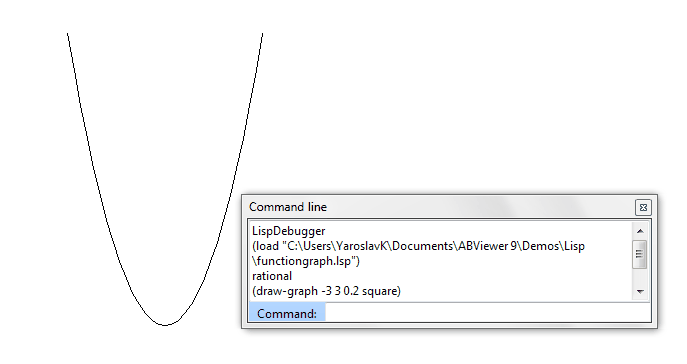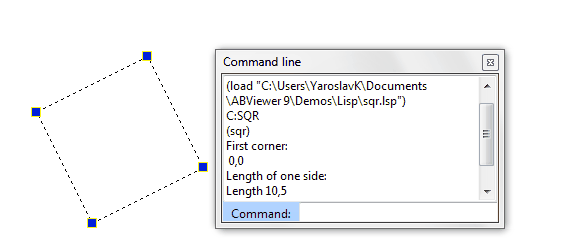﻿ Overview > LISP

# LISP

ABViewer supports about one half of LISP commands (more than 50) including recursive functions and ones that take functions as arguments. If you have worked with LISP, you'll be able to extend ABViewer features by using functions. A built-in debugger is used to debug LISP in ABViewer, you can access it by clicking Advanced > Lisp > Lisp Debugger.

Software package includes two files that demonstrate adding new LISP functions: functiongraph.lsp and sqr.lsp. After the software is installed, the examples are added to the folder c:\Users\USER_NAME\Documents\ABViewer 15\Demos\Lisp\...

functiongraph.lsp

The demo adds the function draw-graph that plots charts.

 (defun draw-line (p1 p2)   (command "_line" p1 p2 ""))   (defun square (x)   (* x x))   (defun rational (x)    (/ 1 x))   (defun draw-graph (min max step f)   (setq x min)   (setq p (list x (f x)))   (while (<= x max)      (draw-line p (setq p (list x (f x))))   (setq x (+ x step))))

To call the function draw-graph in the command line, input:

 (load "С:\Users\USER_NAME\Documents\ ABViewer 15\Demos\Lisp\functiongraph.lsp") Loads the file functiongraph.lsp to the current file (draw-graph -10 10 0.5 square) Plots the function y=x2 (draw-graph -10 10 0.5 sin) Plots the function y=sinx (draw-graph -10 10 0.5 cos) Plots the function y=cosx (draw-graph -10 10 0.5 atan) Plots the function y=arctgx

Here is the result of performing the command:

Command: (draw-graph -10 10 0.5 square)sqr.lsp

Adds the SQR function that draws a square.

 (defun SQR (/ pt1 pt2 pt3 pt4 len ang)   (setq pt1 (getpoint "First corner: "))   (setq pt2 (getpoint "Length of one side: "))   (setq len (distance pt1 pt2))   (setq ang (angle pt1 pt2))   (setq pt3 (polar pt2 (+ ang (/ PI 2.0)) len))   (setq pt4 (polar pt3 (+ ang PI) len))   (command "_PLINE" pt1 pt2 pt3 pt4 pt1 "") )

Here is an example:

 (load "С:\Users\USER_NAME\Documents\ ABViewer 15\Demos\Lisp\sqr.lsp") Loads the file sqr.lsp to the current drawing (sqr) Requests two points the distance between which will be a square side.

Here is the result of performing the commands:

Command: (sqr)

First corner: (0,0)

Length of one side: (10,5)  //the coordinates of the second point were entered instead of the length.LISP Debugger

Go to ABViewer